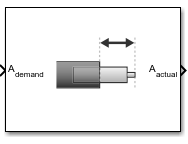# Nonlinear Second-Order Actuator

Implement second-order actuator with rate and deflection limits

• Library:
• Aerospace Blockset / Actuators

•## Description

The Second Order Nonlinear Actuator block outputs the actual actuator position using the input demanded actuator position and other dialog box parameters that define the system.

## Ports

### Input

expand all

Demanded actuator position, specified as a scalar or array.

Data Types: `double`

### Output

expand all

Actual actuator position, returned as a scalar or array.

Data Types: `double`

## Parameters

expand all

Natural frequency of actuator, specified as a scalar double, in radians per second.

#### Programmatic Use

 Block Parameter: `wn_fin` Type: character vector Values: scalar | double Default: `'1'`

Damping ratio of actuator, specified as a scalar double.

#### Programmatic Use

 Block Parameter: `z_fin` Type: character vector Values: scalar | double Default: `'0.3'`

Largest actuator position allowable, specified as a scalar double, in the same units as demanded actuator position.

#### Programmatic Use

 Block Parameter: `fin_max` Type: character vector Values: scalar | double Default: `'20*pi/180'`

Smallest actuator position allowable, specified as a scalar double, in the same units as demanded actuator position.

#### Programmatic Use

 Block Parameter: `fin_min` Type: character vector Values: scalar | double Default: `'-20*pi/180'`

Fastest speed allowable for actuator motion, specified as a scalar double, in the units of demanded actuator position per second.

#### Programmatic Use

 Block Parameter: `fin_maxrate` Type: character vector Values: scalar | double Default: `'500*pi/180'`

Initial position of actuator, specified as a scalar double, in the same units as demanded actuator position.

• If the specified value is less than the value of Minimum deflection, the block sets the value of Minimum deflection as the initial position value.

• If the specified value is greater than the value of Maximum deflection, the block sets the value of Maximum deflection as the initial position value.

#### Programmatic Use

 Block Parameter: `fin_act_0` Type: character vector Values: scalar | double Default: `'0'`

Initial velocity of actuator, specified as a scalar double, in the units of demanded actuator position per second.

If the absolute value of the specified value is greater than the absolute value of Rate Limit, this block sets the value of Rate Limit as the initial velocity value.

#### Programmatic Use

 Block Parameter: `fin_act_vel` Type: character vector Values: scalar | double Default: `'0'`

## Version History

Introduced in R2012a